# College Algebra Tutorial 29

College Algebra
Tutorial 29: CirclesLearning Objectives

After completing this tutorial, you should be able to:
1. Write the equation of a circle in standard form given the radius and center.
2. Write the equation of a circle in standard form given the equation written in general form.
3. Determine what the center and radius of a circle are given the equation of the circle.
4. Graph a circle.Introduction

In this tutorial we get to look at circles.  We will discuss how to write an equation in standard form given either the radius and center or the equation written in general form.  We will revisit the idea of completing the square to help us go from the general form to the standard form of the equation of the circle.  If you need a review on completing the square, feel free to go to Tutorial 17: Quadratic Equations.  We will also look at how to find the center ahd radius of a circle when looking at the equation of it.  And the fun part, we get to graph these circles too.  I guess you better get to it.Tutorial

The Standard Form of the Equation of a Circle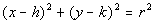(h, k) is the center
(x, y) is any point on the circle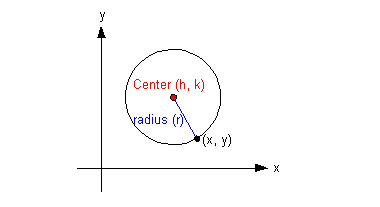All points (x, y) on the circle are a fixed distance (radius) away from the center (h, k).

The h value of your center is the first value of your ordered pair and the k value of your center is the second value of your ordered pair.

We can use this form to plug into when we need to come up with the equation of a circle.

When writing an equation of a circle, keep in mind that you ALWAYS need two pieces of information:

1. The center of the circle.
2. The radius of the circle.
Once you have these two pieces of information, you plug the h and k values from your center and the value of the radius (r) into the standard form of the equation of a circle.Example 1: Write the standard form of the equation of the circle with center (5, 7) and r = 4.

What are the two things we need to write an equation of a circle????
If you said the center and the radius, you are correct.

Looks like we have all the information we need.  We are ready to put our equation together.

What value are we going to replace h with?
If you said 5, you are correct!!!  h is the first number of the ordered pair of the center of the circle.

What value are we going to replace k with?
If you said 7, you are right on!!!  k is the second number of the ordered pair of the center of the circle.

What value are we going to replace r with?
If you said 4, give yourself a pat on the back!!!  r is the radius of the circle.

Putting it into standard form we get: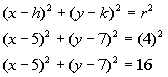*Plug in 5 for h, 7 for k, and 4 for r
*4 squared is 16
*Standard form of circle with center (5, 7) and radius 4Example 2: Write the standard form of the equation of the circle with center (-3, -1) and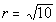.

What are the two things we need to write an equation of a circle????
If you said the center and the radius, you are correct.

Looks like we have all the information we need.  We are ready to put our equation together.

What value are we going to replace h with?
If you said -3, you are correct!!!  h is the first number of the ordered pair of the center of the circle.

What value are we going to replace k with?
If you said -1, you are right on!!!  k is the second number of the ordered pair of the center of the circle.

What value are we going to replace r with?
If you said square root of 10, give yourself a pat on the back!!! r is the radius of the circle.

Putting it into standard form we get:*Plug in -3 for h, -1 for k, and sq. root of 10 for r
*sq. root of 10 squared is 10
*Standard form of circle with center (-3,-1) and radius of sq. root of 10

Make sure that you are careful when one of your values is negative and you have to subtract it as we did in line 2.  x - (-3)  is not the same as x - 3 and y - (-1) is not the same as y - 1.Example 3: Write the standard form of the equation of the circle with center (0, 0) and r = 10.

What are the two things we need to write an equation of a circle????
If you said the center and the radius, you are correct.

Looks like we have all the information we need.  We are ready to put our equation together.

What value are we going to replace h with?
If you said 0, you are correct!!!  h is the first number of the ordered pair of the center of the circle.

What value are we going to replace k with?
If you said 0, you are right on!!!  k is the second number of the ordered pair of the center of the circle.

What value are we going to replace r with?
If you said 10, give yourself a pat on the back!!!  r is the radius of the circle.

Putting it into standard form we get: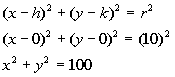*Plug in 0 for h, 0 for k, and 10 for r
*10 squared is 100
*Standard form of circle with center (0, 0) and radius 10

Generally, when you have an expression where you are subtracting 0, you can simplify it by not writing the - 0.  Going from line 2 to 3 above, I simplified the expression x - 0 by writing it in the equivalent form x and then squaring it, it becomes.  Similarly, I wrote y - 0 as y and then squaring it, it becomes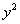.

The General Form of the Equation of a Circle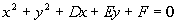of a Circle Given its Equation

Step 1: Write the equation of the circle in standard form ().

If your equation is in standard form it will make it easier for you to identify the center and radius.

If your equation is in the general form you will need to complete the square to both the x terms and the y terms as if they were two separate problems.

Below is a review on completing the square.  If you need more of a review on this topic, feel free to go to Tutorial 17: Quadratic Equations.

Step 1a:  Make sure that the coefficients on theandterms are equal to 1.

If the coefficient ofandare already 1, then proceed to step 1b.

If the coefficient is not equal to 1, then divide both sides by the coefficients of theandsquared terms.

Step 1b:  Isolate the, x,and y terms.

In other words, rewrite it so that the, x,and y terms are all on one side and the constant is on the other  side.

Step 1c:  Complete the square for both x and y.

At this point we will be creating a perfect square trinomial for the x terms as well as the y terms.  Recall that a perfect square trinomial (PST) is of the form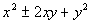and it factors in the form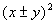.  When it is in that form it will allow us to continue onto the next step and write the equation in standard form,.

We need to find a number that we can add to theand x terms so that we have a PST.  We also need to find a number that we can add to theand y terms so that we have a PST.  We can get that magic number by doing the following:

If we havewe complete its square by adding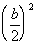to both sides of the equation.

In other words, we complete the square by taking ½ of b (the coefficient of the x term) and then squaring it.  Make sure you remember to add it to BOTH sides to keep the equation balanced.  We do the same type of thing with theand y terms.

Since we will be completing the square for both the x’s and the y’s,  we will have two numbers that we will be adding to both sides.

Step 1d:  Factor both  PST’s created in step 1c as a binomial squared.

This will put the equation of the circle in standard form,.

Step 2: Identify the center and radius of circle.

You can do this by lining up the equation found in step 1 with the standard form of the equation of a circle.

Step 3: Graph, if needed.

This step isn't really part of finding the center or the radius.  But in some cases you will need to graph your circle after finding those two items.  You can graph your circle by plotting your center (h, k) and then using your radius to find points on the circle.  All points (x, y) on the circle are a fixed distance (radius) away from the center (h, k).  Then, draw your circle.

Keep in mind that when we use the letters h and k for our center,  h is the first value of the ordered pair of the center.  In other words, when you graph it, it corresponds to the x-axis. k is the second value of the ordered pair of the center.  In other words, when you graph it, it corresponds to the y-axis.Example 4: Find the center and radius of the circle,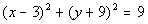, and graph it.

Step 1: Write the equation of the circle in standard form ().

The equation is already in standard form.

Step 2: Identify the center and radius of circle.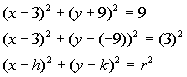*Standard form of circle

*9 is 3 squared

Make sure that you are careful.  The original standard form is, where we have x MINUS h and y MINUS k.  So h is the number that we are subtracting from x and k is the number that we are subtracting from y.  Also, the right side of the equation is r SQUARED

So, in line 2 above, I rewrote the equation to show what numbers we were actually subtracting on the left side of the equation.

On the right side of the equation I showed what number was being squared.  This is to help you see how we get the center (h, k) and the radius (r).

Make sure that you are careful when one of your values is negative and you have to subtract it as we did in line 2.  y - (-9) is not the same as y - 9.

What value is h?
If you said 3, you are correct!!!  Since 3 is the value being subtracted from x in the first (  ), that is the value of h

What value is k?
If you said -9, you are right on!!!  Since -9 is the value being subtracted from y in the second (  ), that is the value of k

What value is r?
If you said 3, give yourself a pat on the back!!!  Since 3 is the number being squared on the right side of the standard form of our equation, that is the value of r.

The center is (3, -9) and the radius is 3.

Step 3: Graph, if needed.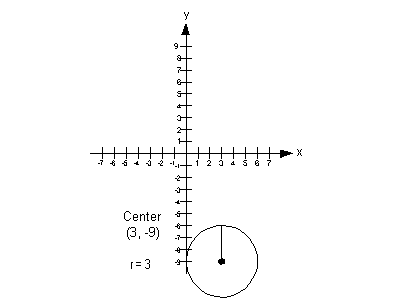Example 5: Find the center and radius of the circle,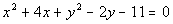, and graph it.

Step 1: Write the equation of the circle in standard form ().

Step 1a:  Make sure that the coefficients on theandterms is equal to 1.

The coefficients on theandterms are both already 1.

Step 1b:  Isolate the, x,and y terms.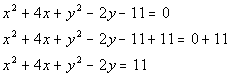*Inverse of sub. 11 is add. 11

Step 1c:  Complete the square for both x and y.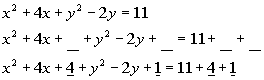*Complete the square for both x and y
*Make sure that you add 4 and 1 to BOTH sides

Completing the square for x (as shown above):
b is the coefficient of the 4x term, which in this case is 4.  Complete the square by taking 1/2 of b and squaring it.  Taking 1/2 of 4 we get 2, and then squaring 2 we get 4.

Completing the square for y (as shown above):
b is the coefficient of the -2y term, which in this case is -2.  Complete the square by taking 1/2 of b and squaring it.  Taking 1/2 of -2 we get -1, and then squaring -1 we get 1.

Step 1d:  Factor both  PST’s created in step 1c as a binomial squared.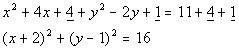*Factor each PST
*Standard form of circle

Step 2: Identify the center and radius of circle.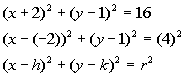*Standard form of circle

*16 is 4 squared

Make sure that you are careful.  The original standard form is, where we have x MINUS h and y MINUS k.  So h is the number that we are subtracting from x and k is the number that we are subtracting from y.  Also, the right side of the equation is r SQUARED

So in line 2 above, I rewrote the equation to show what numbers we were actually subtracting on the left side of the equation.

On the right side of the equation I showed what number was being squared.  This is to help you see how we get the center (h, k) and the radius (r).

Make sure that you are careful when one of your values is negative and you have to subtract it as we did in line 2.  x - (-2)  is not the same as x - 2.

What value is h?
If you said -2, you are correct!!!  Since -2 is the value being subtracted from x in the first (  ), that is the value of h

What value is k?
If you said 1, you are right on!!!  Since 1 is the value being subtracted from y in the second (  ), that is the value of k

What value is r?
If you said 4, give yourself a pat on the back!!!  Since 4 is the number being squared on the right side of the standard form of our equation, that is the value of r.

The center is (-2, 1) and the radius is 4.

Step 3: Graph, if needed.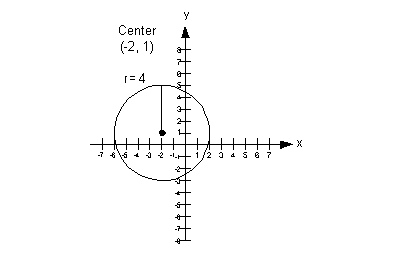Example 6: Find the center and radius of the circle,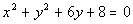, and graph it.

Step 1: Write the equation of the circle in standard form ().

Step 1a:  Make sure that the coefficients on theandterms are equal to 1.

The coefficients on theandterms are both already 1.

Step 1b:  Isolate the, x,and y terms.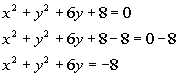*Inverse of add. 8 is sub. 8

Step 1c:  Complete the square for both x and y.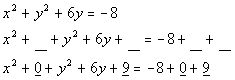*Complete the square for both x and y
*Make sure that you add 0 and 9 to BOTH sides

Completing the square for x (as shown above):
Note how we have an x squared term, but we are missing our x term.  If we are missing a term then it is understood to be 0.  So in this case we would think of our b, the coefficient in front of the x term, as 0.  Taking 1/2 of 0 we get 0, and then squaring 0 we get 0.

Completing the square for y (as shown above):
b is the coefficient of the 6y term, which in this case is 6.  Complete the square by taking 1/2 of b and squaring it.  Taking 1/2 of 6 we get 3, and then squaring 3 we get 9.

Step 1d:  Factor both  PST’s created in step 1c as a binomial squared.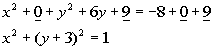*Factor each PST
*Standard form of circle

Note that since we were only adding a 0 to the x squared term, that we can just write that as.

Step 2: Identify the center and radius of circle.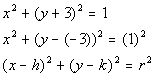*Standard form of circle

*16 is 4 squared

Make sure that you are careful.  The original standard form is, where we have x MINUS h and y MINUS k.  So h is the number that we are subtracting from x and k is the number that we are subtracting from y.  Also, the right side of the equation is r SQUARED

So in line 2 above, I rewrote the equation to show what numbers we were actually subtracting on the left side of the equation.

On the right side of the equation I showed what number was being squared.  This is to help you see how we get the center (h, k) and the radius (r).

Make sure that you are careful when one of your values is negative and you have to subtract it as we did in line 2.  y - (-3)  is not the same as y - 3.

What value is h?
If you said 0, you are correct!!!  Since there is no value being subtracted from x in the first (  ) that means it is understood that 0 is the number being subtracted, that is the value of h

What value is k?
If you said -3, you are right on!!!  Since -3 is the value being subtracted from y in the second (  ), that is the value of k

What value is r?
If you said 1, give yourself a pat on the back!!!  Since 1 is the number being squared on the right side of the standard form of our equation, that is the value of r.

The center is (0, -3) and the radius is 1.

Step 3: Graph, if needed.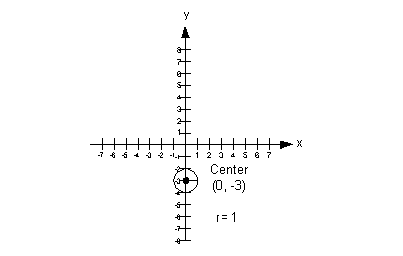Practice Problems

These are practice problems to help bring you to the next level.  It will allow you to check and see if you have an understanding of these types of problems. Math works just like anything else, if you want to get good at it, then you need to practice it.  Even the best athletes and musicians had help along the way and lots of practice, practice, practice, to get good at their sport or instrument.  In fact there is no such thing as too much practice.

To get the most out of these, you should work the problem out on your own and then check your answer by clicking on the link for the answer/discussion for that  problem.  At the link you will find the answer as well as any steps that went into finding that answer.Practice Problems 1a - 1b: Write the standard form of the equation of the circle with the given conditions.

1a. Center (9, -10) and r = 5
1b.  Center (2, 0) and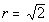Practice Problems 2a - 2b: Find the center and radius of the given circle and graph it.

2a.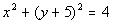2b.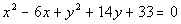Need Extra Help on these Topics?

There are no appropriate web pages to go with these topics.

Go to Get Help Outside the Classroom found in Tutorial 1: How to Succeed in a Math Class for some more suggestions.

Last revised on Feb. 26, 2010 by Kim Seward.